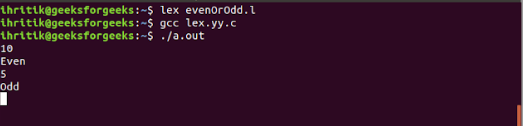# Lex program to check whether a given number is even or odd

• Last Updated : 30 Apr, 2019

Given a number n, the task is to check whether the given n is even or odd using Lex program.

Examples:

```Input : 10
Output : Even

Input : 5
Output : Odd
```

Prerequisite: FLEX (Fast Lexical Analyzer Generator)

An even number is an integer which is “evenly divisible” by 2. This means that if the integer is divided by 2, it yields no remainder or 0 as remainder. Similarly, an Odd number is an integer which is not “evenly divisible” by 2 and will leaves 1 as remainder.

Below is the implementation of above approach:

 `/*Lex program to take check whether``the given number is even or odd */``  ` `%{``#include``int` `i;``%}`` ` `%%`` ` `[0-9]+     {i=``atoi``(yytext);``          ``if``(i%2==0) ``               ``printf``(``"Even"``);``          ``else``         ``printf``(``"Odd"``);}``%%``  ` `int` `yywrap(){}``  ` `/* Driver code */``int` `main()``{``  ` `    ``yylex();``    ``return` `0;``}`

Output:My Personal Notes arrow_drop_up